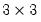Command Reference : Command Reference

 cov
Compute Pearson product-moment (ordinary) covariances for the specified series or groups.
Syntax
cor arg1 [arg2 arg3...]
where arg1, arg2, etc. are the names of series or groups.
Note that this command is a limited feature version of the group view Group::cov.
Options

 wgt=name (optional) Name of series containing weights. wgtmethod=arg (default = “sstdev”) Weighting method (when weights are specified using “weight=”): frequency (“freq”), inverse of variances (“var”), inverse of standard deviation (“stdev”), scaled inverse of variances (“svar”), scaled inverse of standard deviations (“sstdev”). pairwise Compute using pairwise deletion of observations with missing cases (pairwise samples). out=name Basename for saving output. All results will be saved in Sym matrices named using the string “CORR”, appended to the basename (e.g., the correlation specified by “out=my” is saved in the sym matrix “MYCORR”). prompt Force the dialog to appear from within a program. p Print the result.
Examples
cov height weight age
displays aPearson covariance matrix for the three series HEIGHT, WEIGHT, and AGE.
Cross-references
See Group::cov for more general routines for computing covariances.
See also cor .For simple functions to perform the calculations, see @cor, and @cov.